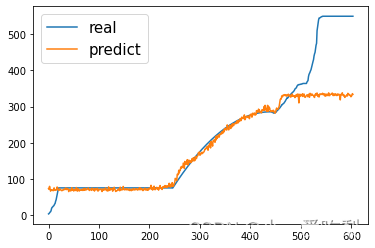# 系列文章目录

 lstm系列文章目录
1.基于pytorch搭建多特征LSTM时间序列预测代码详细解读（附完整代码）
2.基于pytorch搭建多特征CNN-LSTM时间序列预测代码详细解读（附完整代码）

# 二、模型构建

## 1.网络模型构建

def __init__(self,in_channels,out_channels,hidden_size,num_layers,output_size,batch_size,seq_length) -> None:
super(convNet,self).__init__()
self.in_channels=in_channels
self.out_channels=out_channels
self.hidden_size=hidden_size
self.num_layers=num_layers
self.output_size=output_size
self.batch_size=batch_size
self.seq_length=seq_length
self.num_directions=1 # 单向LSTM
self.relu = nn.ReLU(inplace=True)
# (batch_size=64, seq_len=3, input_size=3) ---> permute(0, 2, 1)
# (64, 3, 3)
self.conv=nn.Sequential(
nn.Conv1d(in_channels=self.in_channels, out_channels=self.out_channels, kernel_size=2), #shape(7,--)  ->(64,3,2)
nn.ReLU())
self.lstm=nn.LSTM(input_size=out_channels,hidden_size=hidden_size,num_layers=num_layers,batch_first=True)
self.fc = nn.Linear(hidden_size, output_size)


nn.Conv1d(in_channels, out_channels, kernel_size, stride=1, padding=0, dilation=1, groups=1, bias=True)

'nn.Conv1d(in_channels=self.in_channels, out_channels=self.out_channels, kernel_size=2)

in channels：在文本应用中，即为词向量的维度（在本文中为3,也即特征数） out_channels：卷积产生的通道数，有多少个out_channels，就需要多少个一维卷积（也就是卷积核的数量，在本文中为9）
kernel_size：卷积核的尺寸；卷积核的第二个维度由in_channels决定，所以实际上卷积核的大小为kernel_size * in channels（在本文中为2* 3）
padding：对输入的每一条边，补充0的层数

• 由于是将数据输入至LSTM中，所以数据预处理之后的格式为：

x.shape=(batce_size,seq_length,input_size)

• 将数据输入至网络中，除去relu函数，就是输入至第一层的conv层中，conv层输入格式为：
conv_input=(batch_size,input_size,seq_length),这里注意后两个3交换了

• 经过卷积层卷积后，维度将会变换：
conv_output=(batch_size,output_channels,seq_length-kernel_size+1
这里注释一下，第二个维度为什么从input_size变成了out_channels，因为input_szie就是in_channels，我是为了方便大家从LSTM输入层次理解才写成input_size，卷积层卷积后，in_channels自然就变成了out_channels，这是第二个维度的变换。
第三个维度为什么变成了seq_length-kernel_size+1，这是因为一维卷积中卷积操作是针对seq_len维度进行的，维度可通过公式计算： N = W − F + 2 P S + 1 N=\frac{W-F+2P}{S}+1 。其中：W为输入大小，F为核大小，P为填充大小，S为步长，P=0，S=1，那么维度自然就成了seq_length-kernel_size+1，而这个变换后的维度，会变成下一层LSTM输入维度中的seq_length，这什么意思呢，接下来继续看

• LSTM接收数据形式为input_size=(batce_size,seq_length,input_size)此处input_size=out_channels，将维度交换有：
input_size=(batch_size,seq_length,out_channels)

• LSTM输出结果维度形式为：
output=(batch_size, seq_len, num_directions * hidden_size)

• 全连接层的输入维度形式为：
fc=(hidden_size,output_size)因为是做时间序列预测，这里的output_size直接就是1了

• 展示一下网络结构：
 convNet( (relu): ReLU(inplace=True) (conv): Sequential( (0): Conv1d(3, 9, kernel_size=(2,), stride=(1,)) (1): ReLU() ) (lstm): LSTM(9, 12, num_layers=6, batch_first=True) (fc): Linear(in_features=12, out_features=1, bias=True) )
这就是整个模型数据走下来的流程了，相信这样大家还是能够看到很清晰的，可惜数据最后两个维度都是3，有点混淆但是我也懒得新找数据集了，大家将就一下吧，不懂留言我看见会回的。

## 2.前向传播

def forward(self,x):
x=x.permute(0,2,1)
x=self.conv(x)
x=x.permute(0,2,1)
batch_size, seq_len = x.size(), x.size()
h_0 = torch.randn(self.num_directions * self.num_layers, x.size(0), self.hidden_size)
c_0 = torch.randn(self.num_directions * self.num_layers, x.size(0), self.hidden_size)
# output(batch_size, seq_len, num_directions * hidden_size)
output, _ = self.lstm(x, (h_0, c_0))
pred = self.fc(output)
pred = pred[:, -1, :]
return pred


1. 数据集输入x.shape=(batce_size,seq_length,input_size)=(64,3,3)
2. 卷积层输入conv_input=(batch_size,input_size,seq_length)=(64,3,3)
3. LSTM层输入input_size=(batch_size,seq_length,out_channels)=(64,1,9)

# 三、训练

iter: 4400, loss: 0.00325 iter: 4500, loss: 0.00024 iter: 4600, loss: 0.00368 iter: 4700, loss: 0.0004636.01287
77.402145

216.57518
216.59293

# 总结

cnn-lstm主要是维度和输入限制，建议大家在草稿纸上画一画，会明了很多